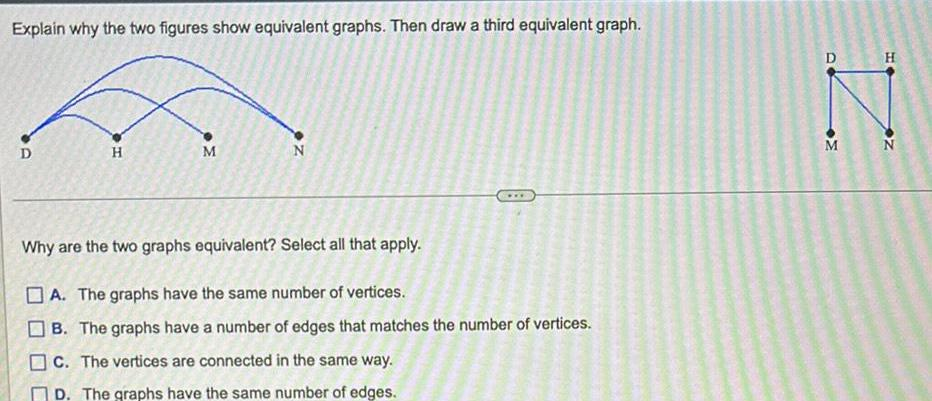Question:

# Explain why the two figures show equivalent graphs Then draw

Last updated: 9/19/2023Explain why the two figures show equivalent graphs Then draw a third equivalent graph D H M M Why are the two graphs equivalent Select all that apply A The graphs have the same number of vertices B The graphs have a number of edges that matches the number of vertices c The vertices are connected in the same way D The graphs have the same number of edges D M H N